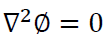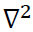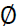### ABSTRACTS FROM INTERNATIONAL CONFERENCE (ICSTSD 2018)

Analysis of Analytical Method of Determining Harmonic Functions
Aung Moe Khaing
Department of Engineering Mathematics
Technological University (Thanlyin)
[email protected]
ABSTRACT
The Laplace’s equation is, whereis the Laplace’s operator andis a scalar function. The solutions of Laplace’s equation are the harmonic functions, which are important in many fields of science and engineering, notably the field of electromagnetism, astronomy, and fluid dynamics, because they can be used to accurately describe the behavior of electric, gravitational, and fluid potentials. We have a problem which is more believable and rigorous analytical method or mathematical analysis to find harmonic function. There are two wide fields in mathematics: field of pure mathematics and field of applied mathematics. In the field of pure mathematics, we use mathematical analysis to prove theorems and formulae. But in the field of applied mathematics, we use techniques and operations to derive analytical methods and to solve sciences and engineering problems. If the sciences and engineering problem is needed to analyze, then these two fields can be used together. In this paper, we present both method and analysis because sometime every problem cannot be solved by using only one method or analysis.
Keywords - Laplace’s equation, harmonic functions, science and engineering, analytical method, mathematical analysis, together, pure mathematics, applied mathematics